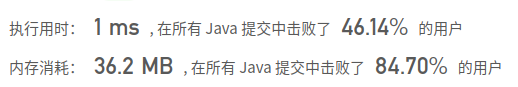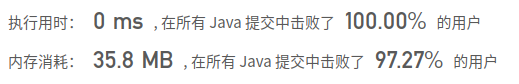# Leetcode: NO.1024 视频拼接 贪心算法

## 题目

示例 1：


示例 2：


示例 3：


示例 4：



1 <= clips.length <= 100
0 <= clips[i] <= clips[i] <= 100
0 <= T <= 100

## 解题记录

• 通过list存储视频出现的起始位置和索引的关系，方便之后查找
• 因为视频一定是从0开始的，那么写一个while循环，第一次找到以0其实的最大值max
• 然后在1到max的之间为起始的，找到这部分中另一端的最大值，如果没有找到更大的还没到T那么说明不可能达到，返回-1
• 如果max大于等于T说明可以，返回count
/**
* @author: ffzs
* @Date: 2020/10/24 上午10:44
*/
public class Solution {

public static int videoStitching(int[][] clips, int T) {
List<List<Integer>> tmp = new ArrayList<>(T+1);
for (int i = 0; i <= T; i++) tmp.add(new ArrayList<>());

for (int i = 0; i < clips.length; i++) {
}

int cur = 0, pre = 0, count=0, max;
while (cur < T) {
max = pre;
for (int i = pre; i <= cur; i++) {
List<Integer> lst = tmp.get(i);
for (Integer integer : lst) {
max = Math.max(clips[integer], max);
}
}
if (max == pre) return -1;
pre = cur+1;
cur = max;
count++;
if (max >= T) return count;
}
return -1;
}
}

class Test {
public static void main(String[] args) {
int[][] clips = {{0,2},{4,6},{8,10},{1,9},{1,5},{5,9}};
//        int[][] clips = {{0,4},{2,8}};
System.out.println(Solution.videoStitching(clips, 10));
}
}• 可能是用了list做一部分数据的缓存归纳，但是对int数据做了包装，会有一定的消耗
• 由于clips长度不大，也可以每次都循环一遍clips，找到适合的起始点
• 由于减少了list的使用，以及装饰过程，时间内存都有优化
/**
* @author: ffzs
* @Date: 2020/10/24 上午11:29
*/
public class Solution2 {
public int videoStitching(int[][] clips, int T) {

int cur = 0, max = 0, count = 0;
while (cur < T) {
for (int[] clip : clips) {
if (clip <= cur) {
max = Math.max(clip, max);
}
}
count++;
if (max >= T) return count;
if (max == cur) return -1;
cur = max;
}
return -1;
}
}06-092万+
07-205万+10-241312
10-2487
10-2443
01-211226
10-2491
10-2432
10-25221